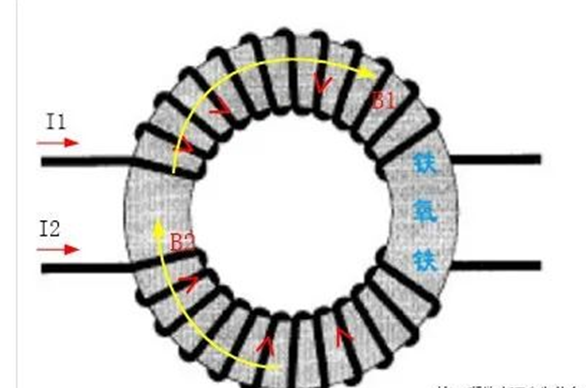# Working principle of common mode choke

17/01/2022
Common mode inductor is a common mode interference suppression device with ferrite as magnetic core. It consists of two coil pairs with the same size and the same number of turns
A four terminal device is wound on the same ferrite ring core to form a large inductance for the common mode signal
It has little effect on the leakage inductance of differential mode signal. The principle is that when a common mode current flows through the magnetic ring
The magnetic flux overlaps with each other, so it has a considerable inductance and suppresses the common mode current
Current, the magnetic flux in the magnetic ring counteracts each other, and there is almost no inductance, so the differential mode current can pass through without attenuation. Therefore, total
The mode inductance can effectively suppress the common mode interference signal in the balanced line, but has no effect on the differential mode signal normally transmitted by the line.
Common mode inductor choke is an important part of switching power supply, frequency converter, UPS power supply and other equipment. Its working principle: when working
When the working current flows through two coils wound in opposite directions, two mutually canceling magnetic fields H1 and H2 are generated. At this time, the working current is mainly affected by
Coil ohmic resistance and negligible damping of small leakage inductance at operating frequency. If an interference signal flows through the coil, the wire
The coil shows high impedance and produces strong damping effect to attenuate the interference signal.## Principle of common mode inductance

Choke is a low impedance coil used to reduce the high-frequency current in the circuit. In order to improve its inductance, the choke usually has a soft magnetic field
The core of material system. The common mode choke has multiple identical coils in which the current flows in reverse, so it is in the core of the choke
The internal magnetic field cancels out. Common mode chokes are often used to suppress interference radiation because such interference currents are reversed in different coils,
Improve the EMC of the system. For such a current, the inductance of the common mode choke is very high. The circuit diagram of common mode inductor is shown in Figure 1.

Common mode signal and differential mode signal are only relative quantities. Common mode signal is also called common mode noise or ground noise, which refers to two lines respectively
For the input filter of switching power supply, the noise to ground is the electrical signal of zero line and live line to the ground respectively. Although the zero line
Neither the zero line nor the live line is directly connected to the earth, but the zero line and the live line can pass through the parasitic capacitance or stray current on the circuit board respectively
Capacitance or parasitic inductance is connected to the earth. Differential mode signal refers to the direct signal difference between two lines, which can also be called TV